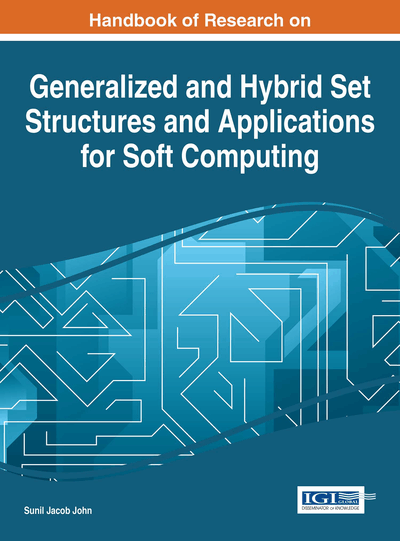# Uncertainty Analysis Using Fuzzy Random Set Theory

Debabrata Datta (Bhabha Atomic Research Centre, India)
DOI: 10.4018/978-1-4666-9798-0.ch019
Available
\$37.50
No Current Special Offers

## Abstract

Uncertainty analysis of any physical model is always an essential task from the point of decision making analysis. Two kinds of uncertainties exist: (1) aleatory uncertainty which is due to randomness of the parameters of models of interest and (2) the epistemic uncertainty which is due to fuzziness of the parameters of the same models. So far both these uncertainties are addressed independently; however since in any practical problem both the types of uncertain variables present, it is required to address them jointly. In order to solve practical problems on uncertainty modeling, it is required to replace the abstract definition of hybrid set by fuzzy random set. Since uncertainty modeling using fuzzy random set has not been carried out so far, the present chapter will address the utility of fuzzy random set for uncertainty modeling on geotechnical and hydrological applications. This chapter will present the fundamentals of fuzzy random set and their application in uncertainty analysis.
Chapter Preview
Top

## 1. Introduction

In any scientific investigation, the relationship between data and theoretical models is very important. Which comes first is not always clear, since the collection, storage and retrieval of large data files is a more or less vague concept. Theoretical models are not expected to represent the data exactly, but at the very least they act as a sorting device that directs the data analyst to efficient ways of extracting information. When a model has a component of randomness in it, there is an extra, although exploitable, source of inexactness (Datta, 2009). By definition, two realizations of the same random phenomenon will not be exactly the same. However, the parameters estimated from two such realizations should be stable. If the realization is large, the the estimates of two parameter become close to each other. The statistical and probabilistic techniques ensure the existence of stochastic models and efficient estimation of their parameters are very well developed for data that are modeled as independent and identically distributed random variables. On the other hand, if any model has a component which is imprecise or vague due to the lack of information or insufficient knowledge of that component, the component is known to have fuzziness or simply the component is fuzzy. Modeling of such quantity is completely different from the component that has randomness. Uncertainty quantification of a model having both these components is a challenging task. Several researchers have expressed their expertise in this direction. For example, uncertainty analysis of a model having random component is generally carried out by Monte Carlo simulation (Binder & Heerman, 1992) with either simple random sampling (SRS) or Latin hypercube sampling (LHS), whereas uncertainty analysis of a model having fuzzy components is carried out by using fuzzy vertex theory (Klir & Yaun, 1995). The uncertainty of a model having random component is categorized as variability or probabilistic or stochastic uncertainty and the uncertainty of a model having fuzzy component is categorized as epistemic uncertainty (Ayyub & Klir, 2006; Datta & Kushwaha, 2009). However, in general, any model for scientific and engineering investigation contains both these components. It is required to make an effort to bring them under same umbrella and then develop a reasonably approximate efficient technique for quantification of the uncertainty of the models. So, what should be that instrument and how we should device that? Soft computing is an important basis for hybridizing these two different platforms as one uncertain component. Before proceeding further, let us investigate as an example the following scenario from the domain of health or environmental risk.

## Complete Chapter List

Search this Book:
Reset